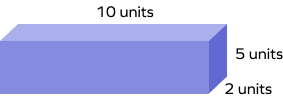# Difference between Cube and Cuboid – Definition, Examples, FAQs

## What Is the Difference between a Cube and a Cuboid?

The major distinction between a cube and a cuboid is that a cube has six identical square faces, while a cuboid has six rectangular faces.

As you can see in the diagram below, the three dimensions: length, width, and height of a cube are the same (a), but that’s not the case with a cuboid.

## Definition of Cube

A cube is a three-dimensional shape with six identical square faces, 8 vertices, and 12 edges. It is a polyhedron. Two adjacent faces of a cube are at right angles to each other. A cube has the same length, width, and height since each face is a square. Each vertex of the cube meets three vertices and three faces.

## Definition of Cuboid

A cuboid is a three-dimensional shape in which there are three pairs of rectangular faces that are opposite and identical. It has 6 quadrilateral faces, 12 edges, and  8 vertices.

A cuboid is also known as a rectangular prism, rectangular box, rectangular parallelepipeds, and right prism.

## Facts about Difference between Cube and Cuboid

• A cube is a three-dimensional solid with all sides of equal length, forming six square faces, while a cuboid is also a three-dimensional solid with six rectangular faces, but its sides can have different lengths.
• Symmetry: A cube has a higher degree of symmetry than a cuboid due to its equal side lengths and identical faces.
• Diagonals: All the diagonals of the cube are equal but a cuboid has equal diagonals for only parallel sides.

## Conclusion

In this article, we explored the fundamental distinctions between cubes and cuboids, understanding their unique properties and applications in geometry. Recognizing these differences aids in problem-solving and real-world context. Let’s apply our knowledge through solving examples and practicing MCQs for better comprehension.

## Solved Examples on Difference Between Cube and Cuboid

1. Find the surface area of a cube given that its sides are equal to 7 units in length.

Solution:

Edge = 7 units

Surface area of cube  = 6 ( edge)2

= 6×72

= 6 × 49

= 294 unit2

Hence, the surface area of the cube is 294 unit2.

2. Find the total surface area of the cuboid with dimensions length = 4 units, breadth =  3 units, and height =  7 units.

Solution:

Given length, breadth, and height = 4, 3, and 7 units, respectively.

Total Surface Area of cuboid = 2 (lb + bh + hl )

= 2 ( 6×5 + 5×9 + 9×6)

= 2 ( 30 + 45 + 54 )

= 2 x 129

= 258 unit2

So, the total surface area of this cuboid is 258 unit2.

3. Find out the volume of a cube if its edge is 7 units.

Solution:

Edge = a = 7 units

The volume of the cube is a3

V = a3

V = 73

V = 343 unit3

The volume of the cube will be 343 unit3.

4. The volume of a rectangular box is 523 unit³ and its base area is 210 unit². Find the height.

Solution:

Volume of the box = (l × b ×h) = 523 unit³

Base Area = (l × b) = 210 unit²

Therefore, the height of the room = (Volume)(Base Area)

h = 523210

h = 2.49 unit

So, the height of the room is 2.49 units.

5. What are the similarities between a cube and a cuboid?

Solution:

Both are 3D shapes.

Both have 6 faces.

Both have 12 edges.

Both have eight vertices.

Both are polyhedrons.

Both have 12 diagonals in total.

## Practice Problems on the Difference between a Cube and a Cuboid

1

### What is the volume of the given cuboid?$60\; feet^{3}$
$100\; feet^{3}$
$90\; feet^{3}$
$50\; feet^{3}$
CorrectIncorrect
Correct answer is: $100\; feet^{3}$
Volume = 10 × 5 × 2 = 100 $feet^{3}$.
2

### A cube has a volume of 125 cubic units. What is the length of each side?

5 units
25 units
375 units
625 units
CorrectIncorrect
V = $side^{3}$ = 125 cubic units
Thus, side = 5 units
3

### The dimensions of a cuboid are l = 5 units, w = 4 units, and h = 3 units. What is its volume?

12 $unit^{3}$
20 $unit^{3}$
60 $unit^{3}$
120 $unit^{3}$
CorrectIncorrect
Correct answer is: 60 $unit^{3}$
V= l × w × h = 5 × 4 × 3 = 60 $unit^{3}$.

## Frequently Asked Questions on the Difference between a Cube and a Cuboid

No, a square is a two-dimensional shape, a quadrilateral with 4 equal sides. A cube is a three-dimensional shape whose each face is a square.

Yes. A cube is a special type of a cuboid, where all the dimensions, such as length, breadth and height, are equal. So, every cube is a cuboid.

Cuboid and cube faces are primarily distinguished by their forms. A cube has a square face, while a cuboid has a rectangle face.

Yes, cubes and cuboids are polyhedrons because they are composed of polygons, such as squares and rectangles.